# Advanced Geometry : How to find the area of a rhombus

## Example Questions

1 2 3 5 Next →

### Example Question #41 : How To Find The Area Of A Rhombus

Find the area of the rhombus.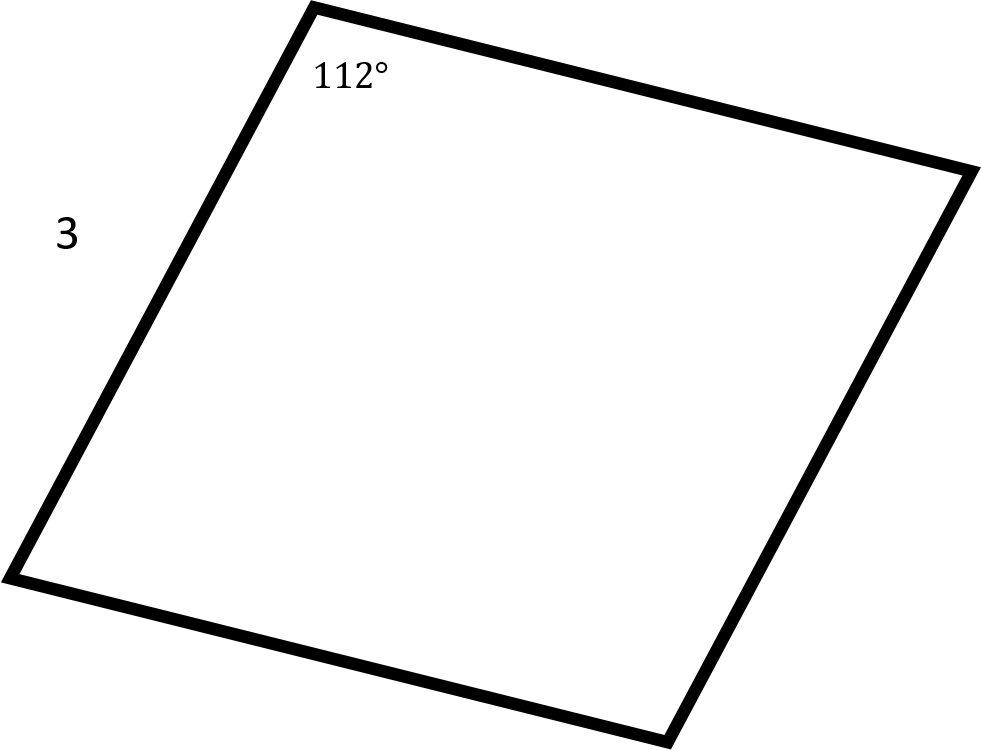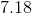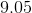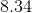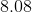Explanation: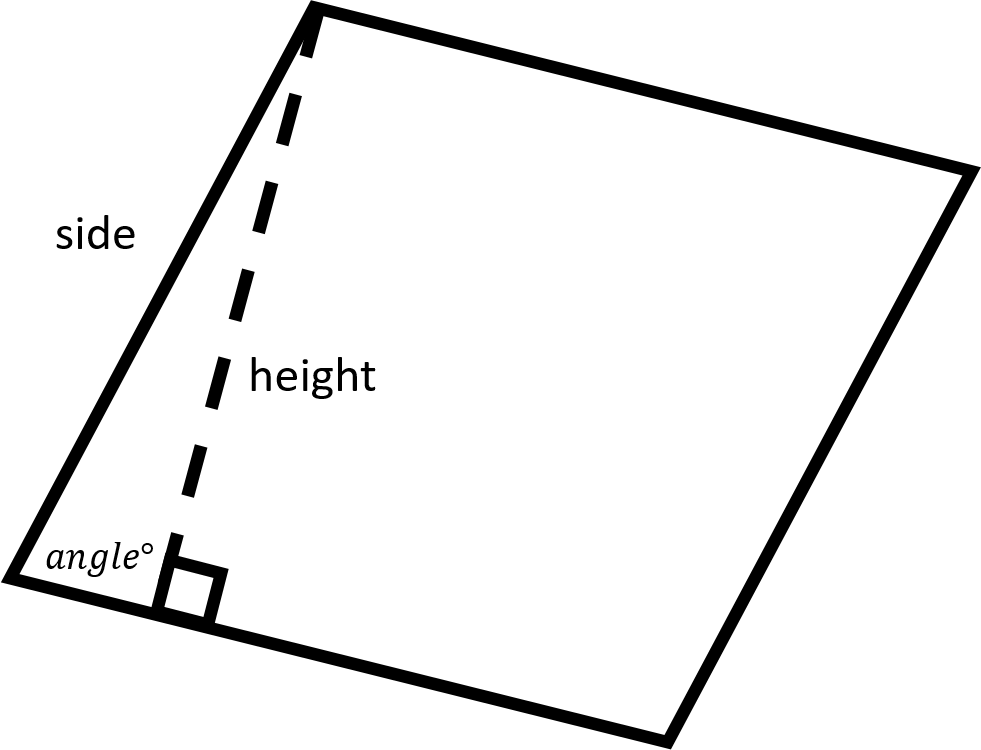Recall that one of the ways to find the area of a rhombus is with the following formula: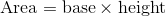Now, since all four sides in the rhombus are the same, we know from the given side value what the length of the base will be. In order to find the length of the height, we will need to use sine.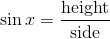, whereis the given angle.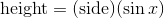Now, plug this into the equation for the area to get the following equation: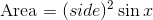Plug in the given side length and angle values to find the area.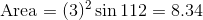Make sure to round toplaces after the decimal.

### Example Question #42 : How To Find The Area Of A Rhombus

Find the area of the rhombus.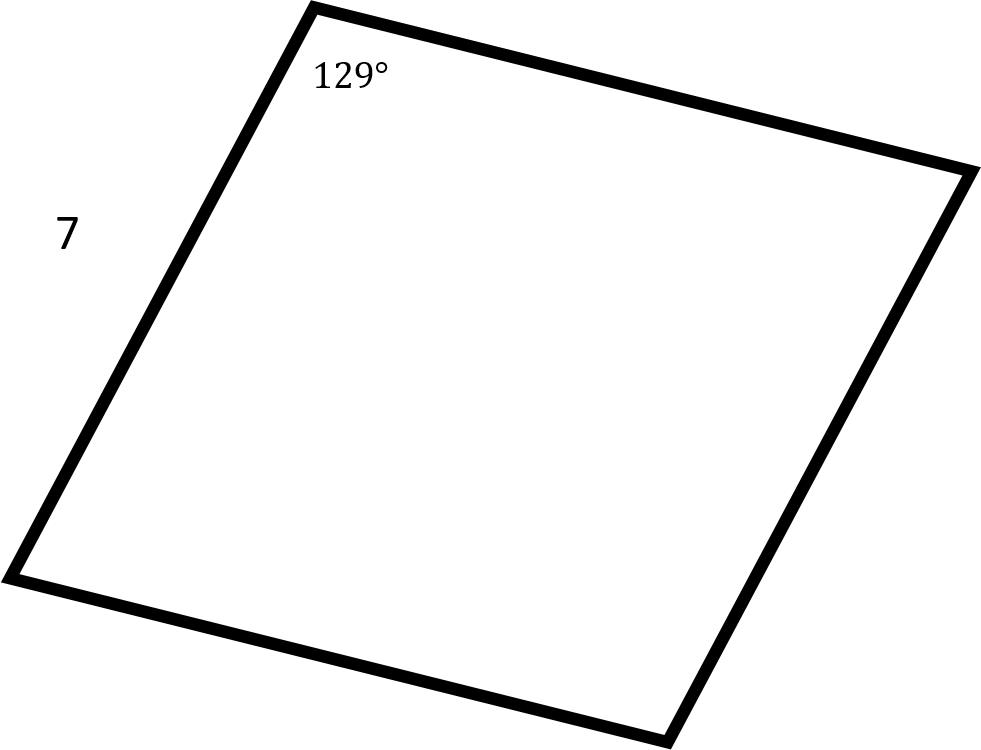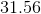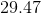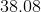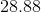Explanation:Recall that one of the ways to find the area of a rhombus is with the following formula:Now, since all four sides in the rhombus are the same, we know from the given side value what the length of the base will be. In order to find the length of the height, we will need to use sine., whereis the given angle.Now, plug this into the equation for the area to get the following equation:Plug in the given side length and angle values to find the area.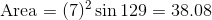Make sure to round toplaces after the decimal.

### Example Question #43 : How To Find The Area Of A Rhombus

Find the area of the rhombus.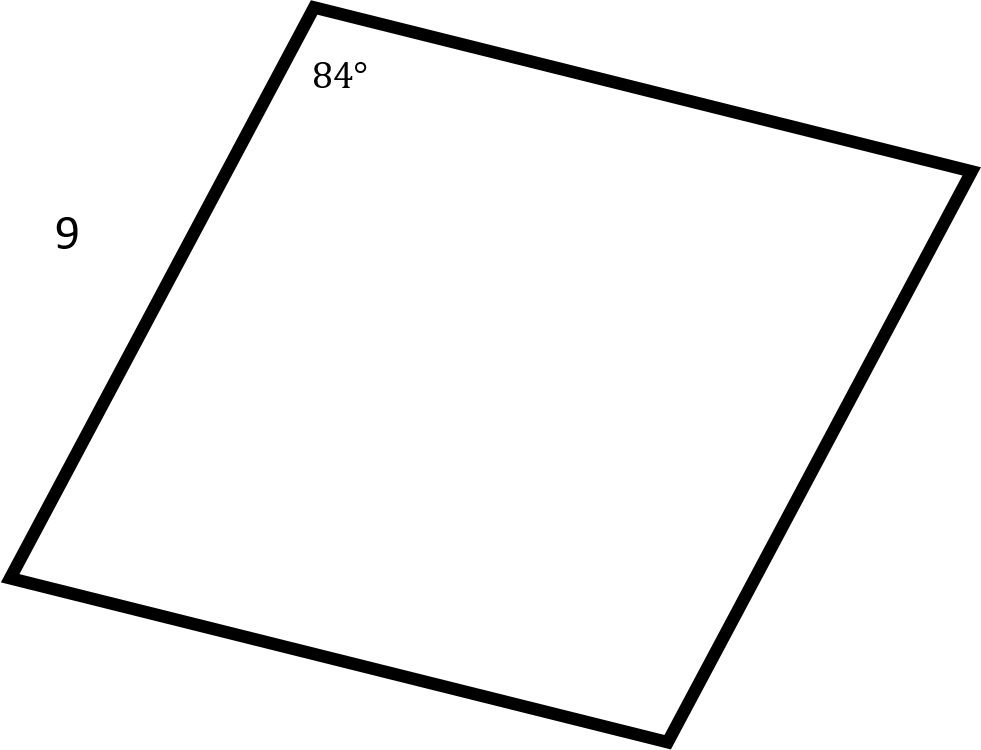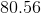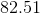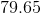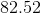Explanation:Recall that one of the ways to find the area of a rhombus is with the following formula:Now, since all four sides in the rhombus are the same, we know from the given side value what the length of the base will be. In order to find the length of the height, we will need to use sine., whereis the given angle.Now, plug this into the equation for the area to get the following equation:Plug in the given side length and angle values to find the area.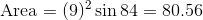Make sure to round toplaces after the decimal.

### Example Question #131 : Quadrilaterals

Find the area of the rhombus.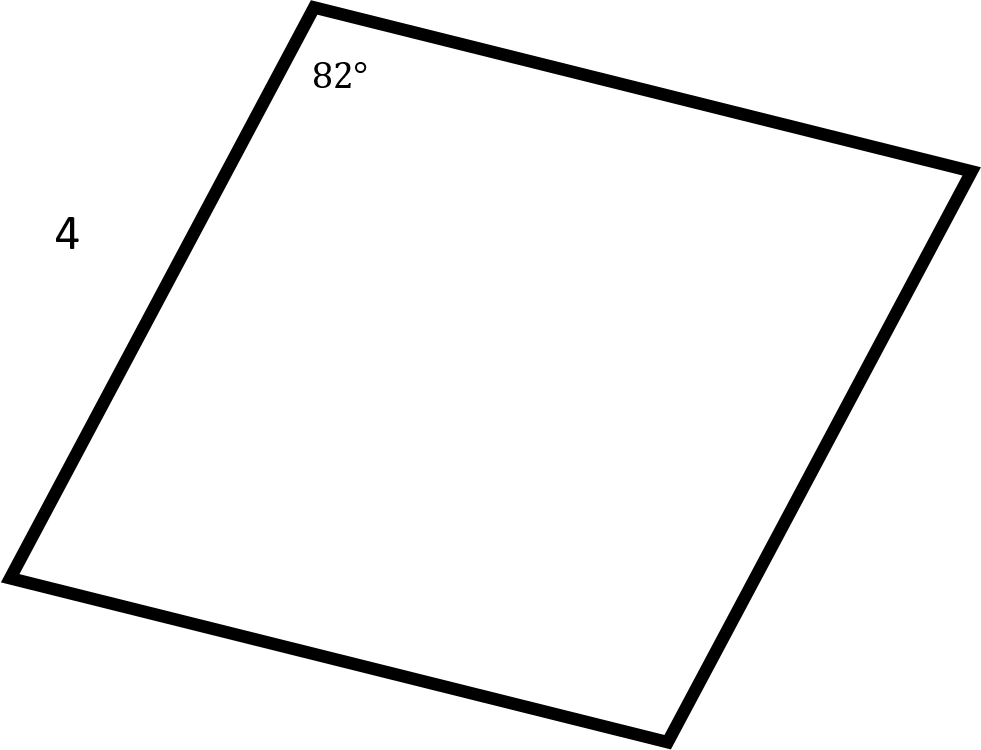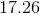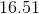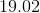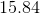Explanation:Recall that one of the ways to find the area of a rhombus is with the following formula:Now, since all four sides in the rhombus are the same, we know from the given side value what the length of the base will be. In order to find the length of the height, we will need to use sine., whereis the given angle.Now, plug this into the equation for the area to get the following equation:Plug in the given side length and angle values to find the area.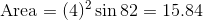Make sure to round toplaces after the decimal.

1 2 3 5 Next →